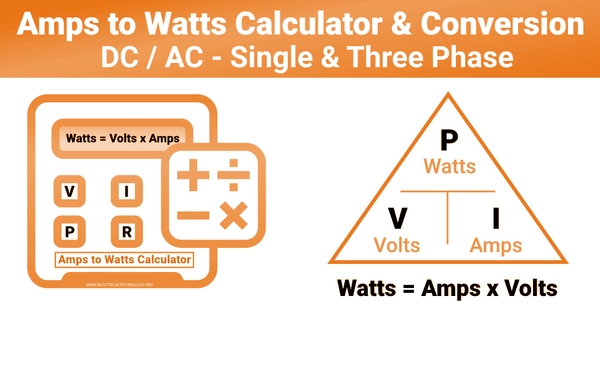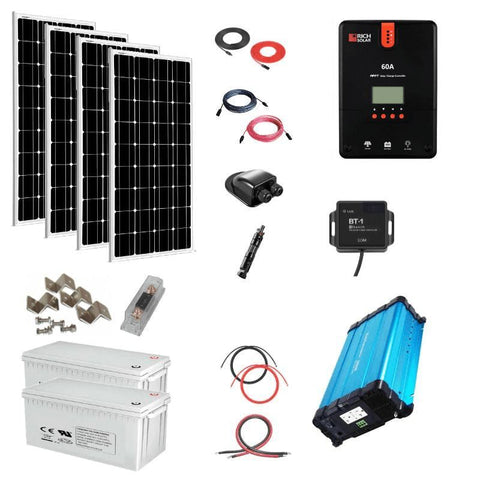DC Watts to AC Watts Conversion Calculator - How-to & Examples - ShopSolar.com

# DC Watts to AC Watts Conversion Calculator

As you probably already know - watts estimate the size of a solar power system.

But does it matter whether they’re in DC (direct current) or AC (alternating current)?

For a variety of reasons, it does, especially when shopping for a solar panel system.

In this article, we explain why understanding DC and AC watts conversions are crucial and show you how to perform conversion calculations.

You might need DC watts to AC watts conversion calculator for the calculations, but it’s not mandatory, as you’ll soon learn.

## What Are DC & AC Watts?

DC watts measure power from current flowing in one direction, whereas AC watts measure electric power from current moving in alternate directions.### DC Watts

DC (direct current) watt is the power solar panels produce. It is also the form of power stored in batteries as their total capacity.

Often, a battery’s capacity is in watt-hours. This requires you to convert watts to watt-hours.

But that’s a discussion for another day. Let's focus on DC to AC conversions today.

In both solar panels and batteries, the DC currents indicate how much power you have to run your appliances.

For solar panels, DC watts come in two ratings:

• Standard Test Conditions (STC) rating – STC is the maximum power rating of a solar panel system, as revealed by the manufacturer.

Calculating the STC rating of solar panels is straightforward, take the number of watts and multiply it by the actual number of solar panels.

For instance, our high-efficiency 330-Watt monocrystalline panel system has 18 – 330 watt solar panels. The STC DC size of this panel system is 5,940 DC Watts (i.e., 330 times 18)

• Performance Test Conditions (PTC) rating – PTC indicates the actual power a solar panel can produce under specific outdoor conditions.

To get this rating, different solar energy regulatory bodies, like the California Energy Commission (CEC), test various solar panels out in the field.

The PTC direct current rating gives a solar panel's power output under ideal weather conditions. Usually, it’s lower than STC ratings.

### AC Watts

On the other hand, AC watt is the form of electric power that runs various equipment, including your household appliances.

For a solar power system to run your home appliances like dishwashers, electric heaters, etc., it must convert the DC power into AC power.

## How to Convert Watts from DC to AC

Most solar equipment use an inverter to convert DC Watts to AC watts. For this reason, you need two things for your DC to AC watts calculations:

• The number of DC watts – it’s always best to use the PTC DC rating because it’s more or less similar to the power output you'll get from a solar panel.

Find the most accurate PTC DC watts for different panels on the California Energy Commission (CEC)’s approved list

• Inverter efficiency rate – the efficiency rates of inverters vary. You can find this information in a product’s description section, on the bottom side, or its specs sheet.

Alternatively, get accurate efficiency rates on CEC’s inverter list.

With the number of watts and inverter efficiency rate, convert DC watts to AC watts using the following formula:

AC watts = (DC watts multiplied by inverter efficiency rate) / 100

For instance: The rating of the monocrystalline panel we mentioned earlier is 330. If we connect the system to an inverter with appx. 90% efficiency rate. The AC watts would be

(330 x 90) / 100 = 297 AC watts

Remember, 297 is the AC wattage for one monocrystalline panel.Since our kit has around 18 panels, multiply 297 by 18 to get the AC watts for the entire system. In this case, you’ll get 5346 AC watts.

Understanding how to convert DC to AC watts is critical for several reasons.

### Why Is DC to AC Watts Conversion Important?

From our calculations, you can confirm power loss occurs during the DC to AC watts conversion process.

We lost about 594 watts (from 5,940 down to 5,346), which translates to around 10% of the initial power output.

Usually, the loss is between 5 to 15% depending on your equipment.

If you do these calculations before purchasing and installing your solar power system, you’ll know how to increase the output and longevity of your system beforehand.

• Choose the right-sized system to match your needs. You’ll be keen to pick the best panels and inverter with a high-efficiency rate to minimize power losses.

You’ll learn how to convert watt-hours to amp hours or convert watt-hours to milliamp hours to cater to all your power needs.

• Install your solar power system correctly – your wiring contributes to the losses in conversions. Knowing your AC needs can guide you to get the correct size wiring for maximum efficiency.
Proper wiring will also increase your system’s life cycle. It will serve you longer without getting damaged.
• Calculate your federal solar tax incentive – federal solar tax credits differ from state to state. But most states will reimburse a specific amount (in dollars) for every AC watt.
• Avoid scams, especially from disingenuous installers – most installers quote a \$ per watt installation fee. Some will use the wrong wattage to charge you more. So if you know how to do these conversions, you’ll always get value for your money.

Alternatively, you can avoid getting scammed by going the DIY route and installing DIY home solar panel kits.## AC to DC Amps Conversion

For these conversions, we recommend using an AC to DC amps calculator. It’s easier and faster. But if you prefer doing the calculations manually, use the following formulas:

• 12 Volt DC Systems

Formula, A 12-volt inverter needs about ten amps of DC input per 100 watts power output used to run an AC load.

For instance: How many DC amps does a 12-volt inverter need to run a 1500-watt electric heater?

The answer: If every 100 watts of output power requires ten amps of input power. What about 1500 watts? Take 1500 times 10, then divide what you'll get by 100

(1500 x 10 = 15,000) / 100 to get 150 amps.

Therefore, 150 amps is the total DC a 12-volt inverter requires to operate a 1,500 ac-watt load.

•     24 Volt DC Systems

Formula, A 24-volt inverter requires around five amps of DC input per 100 watts power output used to run an AC load.

For instance: How many DC amps does a 12-volt inverter need to run the same 1,500-watt electric heater?

Answer: Take 1,500 watts, multiply by 5, then divide by 100

(1,500 x 5 = 7500) / 100 = 75 amps.

A 24-volt inverter needs half (75 amps) of the DC a 12-volt inverter requires to operate the same ac-current load.

Overall, it’s safe to assume that a 24-volt inverter has a higher efficiency rate than a 12-volt inverter. Although there are several factors, like the bulk of your appliances, to consider when choosing the best inverter that can meet your needs without damaging your solar power system.

If you’d rather use these values for your calculations, you can check out our Watts to VA calculator to help you along. There are many different paths that can lead to the same answer, the trick is finding th ones you’re most comfortable taking.## Final Thoughts

Generally, investing in a solar power system is worthwhile, especially if you know how to get the most out of it.

One way to ensure your solar panel system performs at its best for years is to carry out the watts to ac conversions we’ve discussed. And use the info you get to guide your purchase and installation process.

Remember, you can always use the DC watts to AC watts conversion calculator to do the conversions above. All you’ll have to do is fill in the required info in the spaces provided and click the calculate button to get the Ac information.

Did You Find Our Blog Helpful? Then Consider Checking: